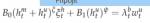janka

New member
Hi all,
I have a problem with solving the following equation. I need to log-linearize it around the steady state so I can use it in DSGE model as a linear equation. However, every time I try to solve it, exponentials xi and phi are always there so, in the end, it's not a linear equation at all. Does anybody know how to solve it and what's the final equation?Thank you!* B_0, B_1, xi and phi are parameters
* b above lambda and every m and u are not exponents, just labels

Last edited: